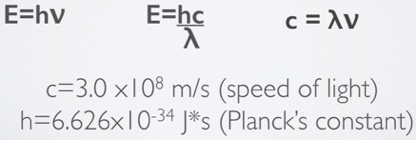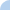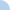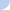# Unit 4.2: Particle Nature of Light Practice

Read all of the following directions BEFORE starting the assignment

The following questions refer to content covered in Unit 4: Notes Video Part 2 (slides #10-16). Write out the work for each of the following wavelength, frequency, and energy calculations within a notebook. You will need a scientific calculator, so if you do not have one you should open one up in a browser. All answers must have the correct number of significant figures and the correct units or they will be marked wrong. You should base significant figures on any measured value given, and not on the constants.

Use the equations and constants below for your calculations.2.7 x 10^-7 m

3.2 x 10^-18 J

1.2 x 10^14 s-1 or 1.2 x 10^14 HzFirst name Last nameThis activity was created by a Quia Web subscriber. Learn more about QuiaCreate your own activities